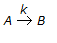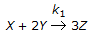# Chemical Engineering - Chemical Reaction Engineering

### Exercise :: Chemical Reaction Engineering - Section 10

36.

B.E.T. method of finding out surface area of a catalyst, uses the extension of __________ isotherm.

 A. Langmuir B. Freundlich C. Tempkin D. none of these

Explanation:

No answer description available for this question. Let us discuss.

37.

In a chemical reaction, repesented by,, it is observed that the
(i) rate of reaction increases by a factor of 4 on doubling the concentration of the reactant.
(ii) rate of reaction increases by a factor of 9 on trebling the concentration of the reactant.
Then the rate of the reaction is proportional to(where, CA = contentration of the reactant)

 A. CA B. CA2 C. CA3 D. CA4

Explanation:

No answer description available for this question. Let us discuss.

38.

Which of the following holds good for an elementary reaction,?

 A. The rate of disappearance of 'Y' is equal to the rate of appearance of 'Z'. B. The rate of disappearance of 'Y' is equal to the rate of disappearance of 'X'. C. Three times the rate of disappearance of 'X' is equal to the rate of appearance of 'Z'. D. The rate of disappearance of 'X' is equal to the rate of appearance of 'Z'.

Explanation:

No answer description available for this question. Let us discuss.

39.

An example of autothermal reactor operation is

 A. sulphur dioxide oxidation B. ethylene oxidation C. benzene oxidation D. ammonia synthesis

Explanation:

No answer description available for this question. Let us discuss.

40.

For a packed bed reactor; the presence of a long tail in the residence time distribution curve is an indication of

 A. ideal plug flow B. bypass C. dead zone D. chanelling